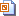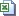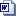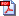Nuffield Mathematics teaching resources are for use in secondary and further education

# Level 2 Algebra and graphs

## Modelling a test drive

Students fit functions to graphs (linear and quadratic). They compare models and comment on their suitability.

This activity is about using graphical and algebraic methods to solve problems in real contexts that can be modelled using quadratic expressions. There are different uses depending on student needs.

## Hire a coach

Introducing linear graphs: this shows how to draw a graph using formulae in Excel. The activities then introduce the concepts of gradient and intercept, and ask students to relate these to the values in the formula and the initial information.Slides (1.2 MB)Graphs Excel 2007 (23 KB)Student 2007 (597 KB)Student 2007 pdf (815 KB)Teacher notes Word (207 KB)Teacher notes pdf (457 KB)

## Goldfish bowl: rearrange formulae

Students practise rearranging formulae. There is a worksheet for individual work, or a set of cards for group work to develop this skill.

## Plumbers' prices

Introduces simultaneous equations and their solution using a graph. The worksheet gives instructions for drawing graphs using formulae in Excel and solving simple simultaneous equations by finding the point of intersection.

## Speed and distance

Students explore the idea that the area under speed-time graphs can be used to find the distance travelled.

Students use acceleration and braking data from a car road test to draw graphs.Students then interpret the graphs and make predictions about values which have not been plotted.

This activity introduces the shape and main features of proportional, linear, inverse proportional, and quadratic graphs.Spreadsheet (220 KB)sheets for Excel 2007 (308 KB)eets for Excel 2007 pdf (775 KB)Teacher notes Word (181 KB)Teacher notes pdf (579 KB)

## Linear graphs

An introduction to linear graphs, leading to the link between the constants in the standard form of the equation (y = mx + c) and the gradient and position of the line.